This example shows you how to use conditional formatting to shade alternate rows. Shading every other row in a range makes it easier to read your data.

1. Select a range.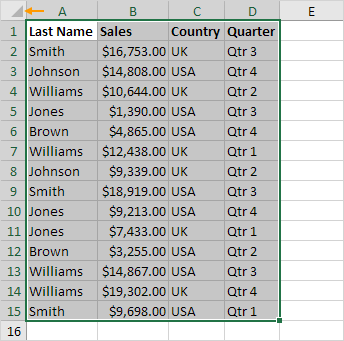2. On the Home tab, in the Styles group, click Conditional Formatting.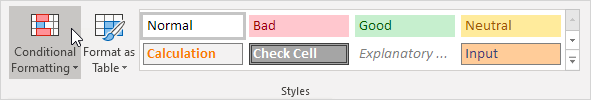3. Click New Rule.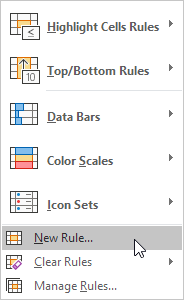4. Select 'Use a formula to determine which cells to format'.

5. Enter the formula =MOD(ROW(),2)

6. Select a formatting style and click OK.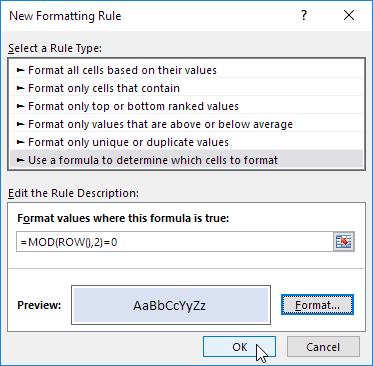Result.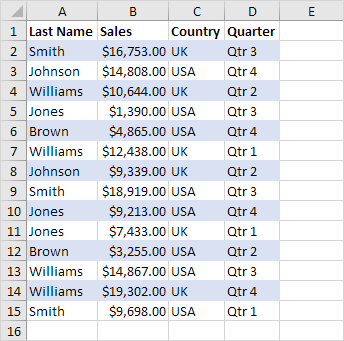Explanation: the MOD function gives the remainder of a division. The ROW() function returns the row number. For example, for the seventh row, MOD(7,2) = 1 because 7 divided by 2 equals 3 with a remainder of 1. For the eight row, MOD(8,2) = 0 because 8 divided by 2 equals 4 with a remainder of 0. As a result, all odd rows return 1 (TRUE) and will be shaded.